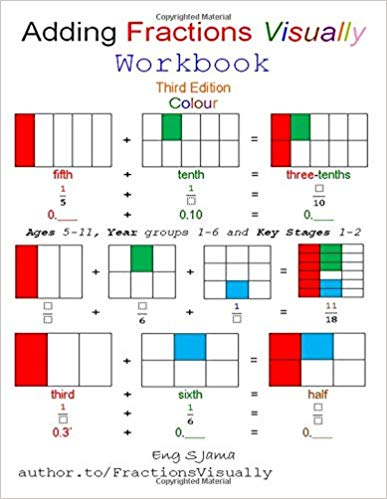# Adding Fractions Visually Workbook : Eng S Jama## Adding Fractions Visually Workbook : Eng S JamaVisual addition of coloured fractions using colour-coded shapes and fraction association.

Wish to master basic maths fractions fast? Look no further!
Fractions Visually is fun, visual and friendly; fraction-tastic way to learn fractions visually. 🙂

For ‘children who think fractions are no fun’, and ‘grown-ups who have never found the best visual resources to master basic maths fractions’.

Excellent introductions to fractions, equivalent fractions and percentages.

Shape, proper fraction, name and decimal of every fraction – from nothing to whole!

Quick quizzes to check learner’s understanding and progress.

Easy language and a lot of illustrations; visual fractions, sums, simple maths vocabulary and many exercises with fully worked examples.

Step-by-step tutorial on adding fractions with same and different denominators.

Adding fractions, equivalent fractions, decimals and percentages.

Superb instructions on finding the Lowest Common Multiple (LCM).

Fill in blank spaces with missing fractions, equivalent fractions, decimal fractions, whole numbers and
percentages.

Fun and visual ways to add fractions visually!

Available as;
Colouring workbook: Adding Fractions Visually: Colouring Workbook.
Paperback: Adding Fractions Visually Third Edition.
Colour paperback: Adding Fractions Visually Third Edition Colour.
Workbook: Adding Fractions Visually Workbook Third Edition.
Colour Workbook (this): Adding Fractions Visually Workbook Third Edition Colour.